# User guide¶

This section includes the machine/trainer guides for learning techniques available in this package.

## Machines¶

Machines are one of the core components of Bob. They represent statistical models or other functions defined by parameters that can be learnt or set by using Trainers.

### K-means machines¶

k-means is a clustering method which aims to partition a set of observations into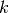clusters. The training procedure is described further below. Here, we explain only how to use the resulting machine. For the sake of example, we create a new bob.learn.misc.KMeansMachine as follows:

>>> machine = bob.learn.misc.KMeansMachine(2,3) # Two clusters with a feature dimensionality of 3
>>> machine.means = numpy.array([[1,0,0],[0,0,1]], 'float64') # Defines the two clusters


Then, given some input data, it is possible to determine to which cluster the data is the closest as well as the min distance.

>>> sample = numpy.array([2,1,-2], 'float64')
>>> print(machine.get_closest_mean(sample)) # Returns the index of the closest mean and the distance to it at the power of 2
(0, 6.0)


### Gaussian machines¶

The bob.learn.misc.Gaussian represents a multivariate diagonal Gaussian (or normal) distribution. In this context, a diagonal Gaussian refers to the covariance matrix of the distribution being diagonal. When the covariance matrix is diagonal, each variable in the distribution is independent of the others.

Objects of this class are normally used as building blocks for more complex bob.learn.misc.GMMMachine or GMM objects, but can also be used individually. Here is how to create one multivariate diagonal Gaussian distribution:

>>> g = bob.learn.misc.Gaussian(2) #bi-variate diagonal normal distribution
>>> g.mean = numpy.array([0.3, 0.7], 'float64')
>>> g.mean
array([ 0.3,  0.7])
>>> g.variance = numpy.array([0.2, 0.1], 'float64')
>>> g.variance
array([ 0.2,  0.1])


Once the bob.learn.misc.Gaussian has been set, you can use it to estimate the log-likelihood of an input feature vector with a matching number of dimensions:

>>> log_likelihood = g(numpy.array([0.4, 0.4], 'float64'))


As with other machines you can save and re-load machines of this type using bob.learn.misc.Gaussian.save() and the class constructor respectively.

### Gaussian mixture models¶

The bob.learn.misc.GMMMachine represents a Gaussian mixture model (GMM), which consists of a mixture of weighted bob.learn.misc.Gaussians.

>>> gmm = bob.learn.misc.GMMMachine(2,3) # Mixture of two diagonal Gaussian of dimension 3


By default, the diagonal Gaussian distributions of the GMM are initialized with zero mean and unit variance, and the weights are identical. This can be updated using the bob.learn.misc.GMMMachine.means, bob.learn.misc.GMMMachine.variances or bob.learn.misc.GMMMachine.weights.

>>> gmm.weights = numpy.array([0.4, 0.6], 'float64')
>>> gmm.means = numpy.array([[1, 6, 2], [4, 3, 2]], 'float64')
>>> gmm.variances = numpy.array([[1, 2, 1], [2, 1, 2]], 'float64')
>>> gmm.means
array([[ 1.,  6.,  2.],
[ 4.,  3.,  2.]])


Once the bob.learn.misc.GMMMachine has been set, you can use it to estimate the log-likelihood of an input feature vector with a matching number of dimensions:

>>> log_likelihood = gmm(numpy.array([5.1, 4.7, -4.9], 'float64'))


As with other machines you can save and re-load machines of this type using bob.learn.misc.GMMMachine.save() and the class constructor respectively.

### Gaussian mixture models Statistics¶

The bob.learn.misc.GMMStats is a container for the sufficient statistics of a GMM distribution.

Given a GMM, the sufficient statistics of a sample can be computed as follows:

>>> gs = bob.learn.misc.GMMStats(2,3)
>>> sample = numpy.array([0.5, 4.5, 1.5])
>>> gmm.acc_statistics(sample, gs)
>>> print(gs)


Then, the sufficient statistics can be accessed (or set as below), by considering the following attributes.

>>> gs = bob.learn.misc.GMMStats(2,3)
>>> log_likelihood = -3. # log-likelihood of the accumulated samples
>>> T = 1 # Number of samples used to accumulate statistics
>>> n = numpy.array([0.4, 0.6], 'float64') # zeroth order stats
>>> sumpx = numpy.array([[1., 2., 3.], [4., 5., 6.]], 'float64') # first order stats
>>> sumpxx = numpy.array([[10., 20., 30.], [40., 50., 60.]], 'float64') # second order stats
>>> gs.log_likelihood = log_likelihood
>>> gs.t = T
>>> gs.n = n
>>> gs.sum_px = sumpx
>>> gs.sum_pxx = sumpxx


### Joint Factor Analysis¶

Joint Factor Analysis (JFA)   is a session variability modelling technique built on top of the Gaussian mixture modelling approach. It utilises a within-class subspace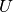, a between-class subspace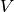, and a subspace for the residuals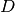to capture and suppress a significant portion of between-class variation.

An instance of bob.learn.misc.JFABase carries information about the matrices,and, which can be shared between several classes. In contrast, after the enrolment phase, an instance of bob.learn.misc.JFAMachine carries class-specific information about the latent variables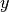and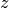.

An instance of bob.learn.misc.JFABase can be initialized as follows, given an existing GMM:

>>> jfa_base = bob.learn.misc.JFABase(gmm,2,2) # dimensions of U and V are both equal to 2
>>> U = numpy.array([[1, 2], [3, 4], [5, 6], [7, 8], [9, 10], [11, 12]], 'float64')
>>> V = numpy.array([[6, 5], [4, 3], [2, 1], [1, 2], [3, 4], [5, 6]], 'float64')
>>> d = numpy.array([0, 1, 0, 1, 0, 1], 'float64')
>>> jfa_base.u = U
>>> jfa_base.v = V
>>> jfa_base.d = d


Next, this bob.learn.misc.JFABase can be shared by several instances of bob.learn.misc.JFAMachine, the initialization being as follows:

>>> m = bob.learn.misc.JFAMachine(jfa_base)
>>> m.y = numpy.array([1,2], 'float64')
>>> m.z = numpy.array([3,4,1,2,0,1], 'float64')


Once the bob.learn.misc.JFAMachine has been configured for a specific class, the log-likelihood (score) that an input sample belongs to the enrolled class, can be estimated, by first computing the GMM sufficient statistics of this input sample, and then calling the bob.learn.misc.JFAMachine.forward() on the sufficient statistics.

>>> gs = bob.learn.misc.GMMStats(2,3)
>>> gmm.acc_statistics(sample, gs)
>>> score = m.forward(gs)


As with other machines you can save and re-load machines of this type using bob.learn.misc.JFAMachine.save() and the class constructor respectively.

### Inter-Session Variability¶

Similarly to Joint Factor Analysis, Inter-Session Variability (ISV) modelling   is another session variability modelling technique built on top of the Gaussian mixture modelling approach. It utilises a within-class subspaceand a subspace for the residualsto capture and suppress a significant portion of between-class variation. The main difference compared to JFA is the absence of the between-class subspace.

Similarly to JFA, an instance of bob.learn.misc.JFABase carries information about the matricesand, which can be shared between several classes, whereas an instance of bob.learn.misc.JFAMachine carries class-specific information about the latent variable.

An instance of bob.learn.misc.ISVBase can be initialized as follows, given an existing GMM:

>>> isv_base = bob.learn.misc.ISVBase(gmm,2) # dimension of U is equal to 2
>>> isv_base.u = U
>>> isv_base.d = d


Next, this bob.learn.misc.ISVBase can be shared by several instances of bob.learn.misc.ISVMachine, the initialization being as follows:

>>> m = bob.learn.misc.ISVMachine(isv_base)
>>> m.z = numpy.array([3,4,1,2,0,1], 'float64')


Once the bob.learn.misc.ISVMachine has been configured for a specific class, the log-likelihood (score) that an input sample belongs to the enrolled class, can be estimated, by first computing the GMM sufficient statistics of this input sample, and then calling the bob.learn.misc.ISVMachine.forward() on the sufficient statistics.

>>> gs = bob.learn.misc.GMMStats(2,3)
>>> gmm.acc_statistics(sample, gs)
>>> score = m.forward(gs)


As with other machines you can save and re-load machines of this type using bob.learn.misc.ISVMachine.save() and the class constructor respectively.

### Total Variability (i-vectors)¶

Total Variability (TV) modelling  is a front-end initially introduced for speaker recognition, which aims at describing samples by vectors of low dimensionality called i-vectors. The model consists of a subspace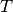and a residual diagonal covariance matrix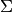, that are then used to extract i-vectors, and is built upon the GMM approach.

An instance of the class bob.learn.misc.IVectorMachine carries information about these two matrices. This can be initialized as follows:

>>> m = bob.learn.misc.IVectorMachine(gmm, 2)
>>> m.t = numpy.array([[1.,2],[4,1],[0,3],[5,8],[7,10],[11,1]])
>>> m.sigma = numpy.array([1.,2.,1.,3.,2.,4.])


Once the bob.learn.misc.IVectorMachine has been set, the extraction of an i-vector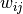can be done in two steps, by first extracting the GMM sufficient statistics, and then estimating the i-vector:

>>> gs = bob.learn.misc.GMMStats(2,3)
>>> gmm.acc_statistics(sample, gs)
>>> w_ij = m.forward(gs)


As with other machines you can save and re-load machines of this type using bob.learn.misc.IVectorMachine.save() and the class constructor respectively.

### Probabilistic Linear Discriminant Analysis (PLDA)¶

Probabilistic Linear Discriminant Analysis   is a probabilistic model that incorporates components describing both between-class and within-class variations. Given a mean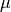, between-class and within-class subspaces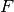and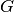and residual noisewith zero mean and diagonal covariance matrix, the model assumes that a sample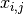is generated by the following process: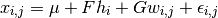Information about a PLDA model (,,and) are carried out by an instance of the class bob.learn.misc.PLDABase.

>>> ### This creates a PLDABase container for input feature of dimensionality 3,
>>> ### and with subspaces F and G of rank 1 and 2 respectively.
>>> pldabase = bob.learn.misc.PLDABase(3,1,2)


Class-specific information (usually from enrollment samples) are contained in an instance of bob.learn.misc.PLDAMachine, that must be attached to a given bob.learn.misc.PLDABase. Once done, log-likelihood computations can be performed.

>>> plda = bob.learn.misc.PLDAMachine(pldabase)
>>> samples = numpy.array([[3.5,-3.4,102], [4.5,-4.3,56]], dtype=numpy.float64)
>>> loglike = plda.compute_log_likelihood(samples)


## Trainers¶

In the previous section, the concept of a machine was introduced. A machine is fed by some input data, processes it and returns an output. Machines can be learnt using trainers in Bob.

### K-means¶

k-means  is a clustering method, which aims to partition a set of observations intoclusters. This is an unsupervised technique. As for PCA , which is implemented in the bob.learn.linear.PCATrainer class, the training data is passed in a 2D numpy.ndarray container.

>>> data = numpy.array([[3,-3,100], [4,-4,98], [3.5,-3.5,99], [-7,7,-100], [-5,5,-101]], dtype='float64')


The training procedure will learn the means for the bob.learn.misc.KMeansMachine. The numberof means is given when creating the machine, as well as the dimensionality of the features.

>>> kmeans = bob.learn.misc.KMeansMachine(2, 3) # Create a machine with k=2 clusters with a dimensionality equal to 3


Then training procedure for k-means is an Expectation-Maximization-based  algorithm. There are several options that can be set such as the maximum number of iterations and the criterion used to determine if the convergence has occurred. After setting all of these options, the training procedure can then be called.

>>> kmeansTrainer = bob.learn.misc.KMeansTrainer()
>>> kmeansTrainer.max_iterations = 200
>>> kmeansTrainer.convergence_threshold = 1e-5

>>> kmeansTrainer.train(kmeans, data) # Train the KMeansMachine
>>> print(kmeans.means)
[[ -6.   6.  -100.5]
[  3.5 -3.5   99. ]]


### Maximum likelihood for Gaussian mixture model¶

A Gaussian mixture model (GMM)  is a common probabilistic model. In order to train the parameters of such a model it is common to use a maximum-likelihood (ML) approach . To do this we use an Expectation-Maximization (EM) algorithm . Let’s first start by creating a bob.learn.misc.GMMMachine. By default, all of the Gaussian’s have zero-mean and unit variance, and all the weights are equal. As a starting point, we could set the mean to the one obtained with k-means .

>>> gmm = bob.learn.misc.GMMMachine(2,3) # Create a machine with 2 Gaussian and feature dimensionality 3
>>> gmm.means = kmeans.means # Set the means to the one obtained with k-means


The Bob class to learn the parameters of a GMM  using ML  is bob.learn.misc.ML_GMMTrainer. It uses an EM-based  algorithm and requires the user to specify which parameters of the GMM are updated at each iteration (means, variances and/or weights). In addition, and as for k-means , it has parameters such as the maximum number of iterations and the criterion used to determine if the parameters have converged.

>>> trainer = bob.learn.misc.ML_GMMTrainer(True, True, True) # update means/variances/weights at each iteration
>>> trainer.convergence_threshold = 1e-5
>>> trainer.max_iterations = 200
>>> trainer.train(gmm, data)
>>> print(gmm)


### MAP-adaptation for Gaussian mixture model¶

Bob also supports the training of GMMs  using a maximum a posteriori (MAP) approach . MAP is closely related to the ML  technique but it incorporates a prior on the quantity that we want to estimate. In our case, this prior is a GMM . Based on this prior model and some training data, a new model, the MAP estimate, will be adapted.

Let’s consider that the previously trained GMM  is our prior model.

>>> print(gmm)


The training data used to compute the MAP estimate  is again stored in a 2D numpy.ndarray container.

>>> dataMAP = numpy.array([[7,-7,102], [6,-6,103], [-3.5,3.5,-97]], dtype='float64')


The Bob class used to perform MAP adaptation training  is bob.learn.misc.MAP_GMMTrainer. As with the ML estimate , it uses an EM-based  algorithm and requires the user to specify which parts of the GMM are adapted at each iteration (means, variances and/or weights). In addition, it also has parameters such as the maximum number of iterations and the criterion used to determine if the parameters have converged, in addition to this there is also a relevance factor which indicates the importance we give to the prior. Once the trainer has been created, a prior GMM  needs to be set.

>>> relevance_factor = 4.
>>> trainer = bob.learn.misc.MAP_GMMTrainer(relevance_factor, True, False, False) # mean adaptation only
>>> trainer.convergence_threshold = 1e-5
>>> trainer.max_iterations = 200
>>> trainer.set_prior_gmm(gmm)
True
>>> gmmAdapted = bob.learn.misc.GMMMachine(2,3) # Create a new machine for the MAP estimate


### Joint Factor Analysis¶

The training of the subspace,andof a Joint Factor Analysis model, is performed in two steps. First, GMM sufficient statistics of the training samples should be computed against the UBM GMM. Once done, we get a training set of GMM statistics:

>>> F1 = numpy.array( [0.3833, 0.4516, 0.6173, 0.2277, 0.5755, 0.8044, 0.5301, 0.9861, 0.2751, 0.0300, 0.2486, 0.5357]).reshape((6,2))
>>> F2 = numpy.array( [0.0871, 0.6838, 0.8021, 0.7837, 0.9891, 0.5341, 0.0669, 0.8854, 0.9394, 0.8990, 0.0182, 0.6259]).reshape((6,2))
>>> F=[F1, F2]

>>> N1 = numpy.array([0.1379, 0.1821, 0.2178, 0.0418]).reshape((2,2))
>>> N2 = numpy.array([0.1069, 0.9397, 0.6164, 0.3545]).reshape((2,2))
>>> N=[N1, N2]

>>> gs11 = bob.learn.misc.GMMStats(2,3)
>>> gs11.n = N1[:,0]
>>> gs11.sum_px = F1[:,0].reshape(2,3)
>>> gs12 = bob.learn.misc.GMMStats(2,3)
>>> gs12.n = N1[:,1]
>>> gs12.sum_px = F1[:,1].reshape(2,3)

>>> gs21 = bob.learn.misc.GMMStats(2,3)
>>> gs21.n = N2[:,0]
>>> gs21.sum_px = F2[:,0].reshape(2,3)
>>> gs22 = bob.learn.misc.GMMStats(2,3)
>>> gs22.n = N2[:,1]
>>> gs22.sum_px = F2[:,1].reshape(2,3)

>>> TRAINING_STATS = [[gs11, gs12], [gs21, gs22]]


In the following, we will allocate a bob.learn.misc.JFABase machine, that will then be trained.

 >>> jfa_base = bob.learn.misc.JFABase(gmm, 2, 2) # the dimensions of U and V are both equal to 2


Next, we initialize a trainer, which is an instance of bob.learn.misc.JFATrainer, as follows:

>>> jfa_trainer = bob.learn.misc.JFATrainer(10) # 10 is the number of iterations


The training process is started by calling the bob.learn.misc.JFATrainer.train().

>>> jfa_trainer.train(jfa_base, TRAINING_STATS)


Once the training is finished (i.e. the subspaces,andare estimated), the JFA model can be shared and used by several class-specific models. As for the training samples, we first need to extract GMM statistics from the samples. These GMM statistics are manually defined in the following.

>>> Ne = numpy.array([0.1579, 0.9245, 0.1323, 0.2458]).reshape((2,2))
>>> Fe = numpy.array([0.1579, 0.1925, 0.3242, 0.1234, 0.2354, 0.2734, 0.2514, 0.5874, 0.3345, 0.2463, 0.4789, 0.5236]).reshape((6,2))
>>> gse1 = bob.learn.misc.GMMStats(2,3)
>>> gse1.n = Ne[:,0]
>>> gse1.sum_px = Fe[:,0].reshape(2,3)
>>> gse2 = bob.learn.misc.GMMStats(2,3)
>>> gse2.n = Ne[:,1]
>>> gse2.sum_px = Fe[:,1].reshape(2,3)
>>> gse = [gse1, gse2]


Class-specific enrollment can then be perfomed as follows. This will estimate the class-specific latent variablesand:

>>> m = bob.learn.misc.JFAMachine(jfa_base)
>>> jfa_trainer.enrol(m, gse, 5) # where 5 is the number of enrollment iterations


### Inter-Session Variability¶

The training of the subspaceandof an Inter-Session Variability model, is performed in two steps. As for JFA, GMM sufficient statistics of the training samples should be computed against the UBM GMM. Once done, we get a training set of GMM statistics. Next, we will allocate an bob.learn.misc.ISVBase machine, that will then be trained.

 >>> isv_base = bob.learn.misc.ISVBase(gmm, 2) # the dimensions of U is equal to 2


Next, we initialize a trainer, which is an instance of bob.learn.misc.ISVTrainer, as follows:

>>> isv_trainer = bob.learn.misc.ISVTrainer(10, 4.) # 10 is the number of iterations, and 4 is the relevance factor


The training process is started by calling the bob.learn.misc.ISVTrainer.train().

>>> isv_trainer.train(isv_base, TRAINING_STATS)


Once the training is finished (i.e. the subspacesandare estimated), the ISV model can be shared and used by several class-specific models. As for the training samples, we first need to extract GMM statistics from the samples. Class-specific enrollment can then be perfomed, which will estimate the class-specific latent variable:

>>> m = bob.learn.misc.ISVMachine(isv_base)
>>> isv_trainer.enrol(m, gse, 5) # where 5 is the number of iterations


### Total Variability (i-vectors)¶

The training of the subspaceandof a Total Variability model, is performed in two steps. As for JFA and ISV, GMM sufficient statistics of the training samples should be computed against the UBM GMM. Once done, we get a training set of GMM statistics. Next, we will allocate an instance of bob.learn.misc.IVectorMachine, that will then be trained.

 >>> m = bob.learn.misc.IVectorMachine(gmm, 2)
>>> m.variance_threshold = 1e-5


Next, we initialize a trainer, which is an instance of bob.learn.misc.IVectorTrainer, as follows:

>>> ivec_trainer = bob.learn.misc.IVectorTrainer(update_sigma=True, max_iterations=10)
>>> TRAINING_STATS_flatten = [gs11, gs12, gs21, gs22]


The training process is started by calling the bob.learn.misc.IVectorTrainer.train().

>>> ivec_trainer.train(m, TRAINING_STATS_flatten)


### Probabilistic Linear Discriminant Analysis (PLDA)¶

Probabilistic Linear Discriminant Analysis  is a probabilistic model that incorporates components describing both between-class and within-class variations. Given a mean, between-class and within-class subspacesandand residual noisewith zero mean and diagonal covariance matrix, the model assumes that a sampleis generated by the following process:An Expectaction-Maximization algorithm can be used to learn the parameters of this model,and. As these parameters can be shared between classes, there is a specific container class for this purpose, which is bob.learn.misc.PLDABase. The process is described in detail in .

Let us consider a training set of two classes, each with 3 samples of dimensionality 3.

>>> data1 = numpy.array([[3,-3,100], [4,-4,50], [40,-40,150]], dtype=numpy.float64)
>>> data2 = numpy.array([[3,6,-50], [4,8,-100], [40,79,-800]], dtype=numpy.float64)
>>> data = [data1,data2]


Learning a PLDA model can be performed by instantiating the class bob.learn.misc.PLDATrainer, and calling the bob.learn.misc.PLDATrainer.train() method.

>>> ### This creates a PLDABase container for input feature of dimensionality 3,
>>> ### and with subspaces F and G of rank 1 and 2 respectively.
>>> pldabase = bob.learn.misc.PLDABase(3,1,2)

>>> trainer = bob.learn.misc.PLDATrainer()
>>> trainer.train(pldabase, data)


Once trained, this PLDA model can be used to compute the log-likelihood of a set of samples given some hypothesis. For this purpose, a bob.learn.misc.PLDAMachine should be instantiated. Then, the log-likelihood that a set of samples share the same latent identity variable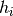(i.e. the samples are coming from the same identity/class) is obtained by calling the bob.learn.misc.PLDAMachine.compute_log_likelihood() method.

>>> plda = bob.learn.misc.PLDAMachine(pldabase)
>>> samples = numpy.array([[3.5,-3.4,102], [4.5,-4.3,56]], dtype=numpy.float64)
>>> loglike = plda.compute_log_likelihood(samples)


If separate models for different classes need to be enrolled, each of them with a set of enrolment samples, then, several instances of bob.learn.misc.PLDAMachine need to be created and enroled using the bob.learn.misc.PLDATrainer.enrol() method as follows.

>>> plda1 = bob.learn.misc.PLDAMachine(pldabase)
>>> samples1 = numpy.array([[3.5,-3.4,102], [4.5,-4.3,56]], dtype=numpy.float64)
>>> trainer.enrol(plda1, samples1)
>>> plda2 = bob.learn.misc.PLDAMachine(pldabase)
>>> samples2 = numpy.array([[3.5,7,-49], [4.5,8.9,-99]], dtype=numpy.float64)
>>> trainer.enrol(plda2, samples2)


Afterwards, the joint log-likelihood of the enrollment samples and of one or several test samples can be computed as previously described, and this separately for each model.

>>> sample = numpy.array([3.2,-3.3,58], dtype=numpy.float64)
>>> l1 = plda1.compute_log_likelihood(sample)
>>> l2 = plda2.compute_log_likelihood(sample)


In a verification scenario, there are two possible hypotheses: 1.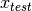and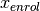share the same class. 2.andare from different classes. Using the methods bob.learn.misc.PLDAMachine.forward() or bob.learn.misc.PLDAMachine.__call__() function, the corresponding log-likelihood ratio will be computed, which is defined in more formal way by: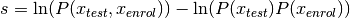>>> s1 = plda1(sample)
>>> s2 = plda2(sample)

  (1, 2, 3, 4, 5, 6) http://en.wikipedia.org/wiki/Mixture_model
  (1, 2, 3, 4) http://en.wikipedia.org/wiki/Maximum_likelihood